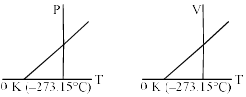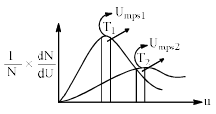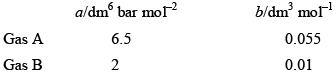Courses

# Test: Gaseous State - 6

## 30 Questions MCQ Test Physical Chemistry | Test: Gaseous State - 6

Description
This mock test of Test: Gaseous State - 6 for Chemistry helps you for every Chemistry entrance exam. This contains 30 Multiple Choice Questions for Chemistry Test: Gaseous State - 6 (mcq) to study with solutions a complete question bank. The solved questions answers in this Test: Gaseous State - 6 quiz give you a good mix of easy questions and tough questions. Chemistry students definitely take this Test: Gaseous State - 6 exercise for a better result in the exam. You can find other Test: Gaseous State - 6 extra questions, long questions & short questions for Chemistry on EduRev as well by searching above.
QUESTION: 1

Solution:
QUESTION: 2

Solution:
QUESTION: 3

### According to kinetic theory of gases, for a diatomic molecule:

Solution:

According to the kinetic molecular theory, the average kinetic energy of gas particles is proportional to the absolute temperature of the gas. This can be expressed with the following equation where k represents the Boltzmann constant.

QUESTION: 4

The ratio between the rms velocity of H2 at 50 K and that of O2 at 800 K is:

Solution:

The formula used for root mean square speed is:

vrms = (3RT/M)1/2

where, vrms = root mean square speed

R = gas constant

T = temperature = 50 K

M = atomic mass of hydrogen = 2 g/mole

Now put all the given values in the above root mean square speed formula, we get:

vrms = [(3*R*50)/2]1/2

2) For rms of Oat 800 K.

vrms = (3RT/M)1/2

where,

vrms = root mean square speed

R = gas constant

T = temperature =800 K

M = atomic mass of oxygen = 32 g/mole

Now put all the given values in the above root mean square speed formula, we get:

vrms = [(3*R*800)/2]1/2

The ratio between the rms speed of H2 at 50K and that of O2 at 800K is

[(3*R*50)/2]1/2/[(3*R*800)/2]1/2

= 1

QUESTION: 5

The compressibility factor for an ideal gas is:

Solution:

It may be thought of as the ratio of the actual volume of a real gas to the volume predicted by the ideal gas at the same temperature and pressure as the actual volume. For an ideal gas, Z always has a value of 1.

QUESTION: 6

X mL of H2 gas effuses through a hole in a container in 5 seconds. The time taken for the effusion of the same volume of the gas specified below under identical conditions is:

Solution:
QUESTION: 7

One mole of N2O4(g) at 300 K is kept in a closed container under one atmosphere. It is heated to 600 K when 20% by mass of N2O4(g) decomposes to NO2(g). The resultant pressure is:

Solution:
QUESTION: 8

When a gas is passed through a small hole at a temperature greater then its critical temperature, Joule-Thomson effect will show:

Solution:
QUESTION: 9

Which of the following choice(s) is (are) correct for a gas:

Solution:
QUESTION: 10

The average velocity of anideal gas molecule at 27°C is 0.3 m/s. The average velocity at 927°C will be:

Solution:

We know,
V1/V2 = [T1/T2]1/2
T1 = 27o
= 300K
T2 = 927o
= 1200K
V1 = 0.3ms-1
V2 = [T2/T1]1/2 × V1

= [1200/300] × 0.3
= 0.6ms-1

QUESTION: 11

Which of the fo llowing is/are correct?

(I) If liquid “kryptonite” (from Superman’s home planet), with a density twice that of mercury, is used in a barometer on earth, one standard atmosphere of pressure would support a column of mercury 380 mm in height.
(II) At constant volume and number of moles of gas, the pressure exhibited by an ideal gas is directly proportional to its absolute temperature.
(III) At standard temperature and pressure (STP), the volume of a mixture of gases containing 0.400 mol H2, 0.600 mo l N2, and 1.00 mol of O2 is 44.8 L and the mole fraction of N2 is 0.300.

Solution:
QUESTION: 12

Which of the following is(are) true for the real gases. (‘C’ represents so me constant value, ‘R’ represents molar gas constant).

Solution:
QUESTION: 13

Which of the following statements are correct?

Solution:
QUESTION: 14

What conclusion would you draw from the following graphs for an ideal gas:Solution:
QUESTION: 15

At Boyle temperature:

Solution:
QUESTION: 16

Indicate the correct statement for equal volume of N2(g) and CO2(g) at 25°C and 1 atm:

Solution:
QUESTION: 17

Which of the following is correct for critical temperature:

Solution:
QUESTION: 18

Consider the following statement regarding Maxwell’s distribution of velocities. The correct statement(s) is/are:

Solution:
QUESTION: 19

If a gas expands at a constant pressure by providing heat:

Solution:
QUESTION: 20

Select incorrect statement(s):

Solution:
QUESTION: 21

Following represents the Maxwell distribution curve for an ideal gas at two temperature T1 and T2. Which of the following option(s) are true?Solution:
QUESTION: 22

Select the correct statement(s):

Solution:
QUESTION: 23

Select the correct statement:

Solution:
QUESTION: 24

Select incorrect statements for real gas:

Solution:
QUESTION: 25

Select correct statements:

Solution:
QUESTION: 26

Which graphs represent the deviation fro mideal gas for H2:

Solution:
QUESTION: 27

Select incorrect statement(s):

Solution:
QUESTION: 28

If an ideal gas is heated at constant pressure:

Solution:
QUESTION: 29

Select correct statement:

Solution:
QUESTION: 30

Consider the following statements. If the van der Waal’s parameters of two gases are given asThen:

Solution: